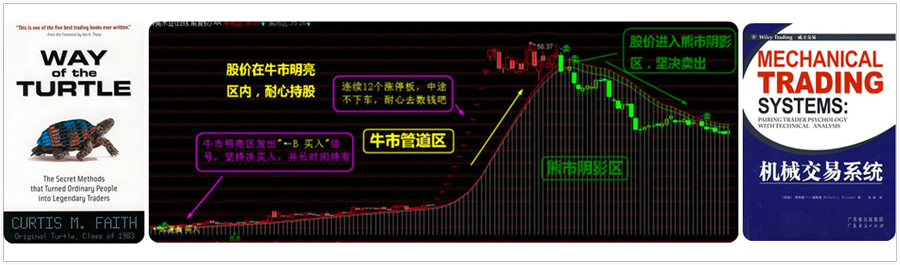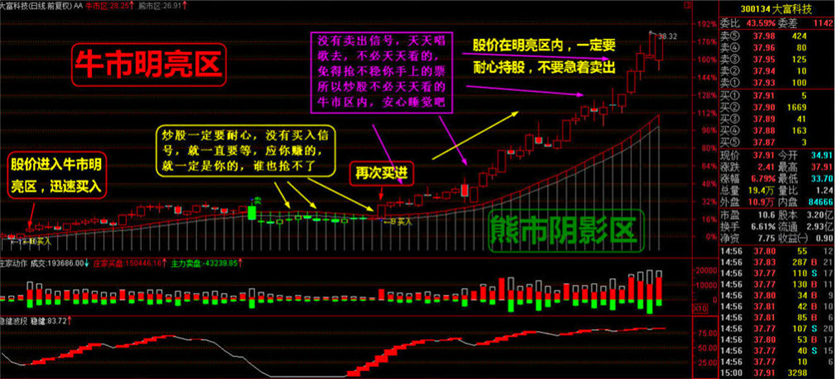# 大智慧经典指标公式多空资金股票软件副图公式源码

2019-02-10 17:06:44  阅读 715 次 评论 0 条N:=30;N2:=25;

Var1:=1;

Var2:=AMOUNT/VOL/100;
Var3:=(EMA(Var2,3)+EMA(Var2,6)+EMA(Var2,12)+EMA(Var2,24))/4;
Var4:=MA(CLOSE,N);
Var5:=MA(CLOSE,N2);
Var6:=Var5+2.6*STDP(CLOSE,N);

STICKLINE(多方资金>空方资金,-5,0,2,1), , ;
STICKLINE(多方资金<空方资金,-5,0,0.8,1), , ;
-8;
-5;
DRAWICON(CROSS(多方资金,空方资金),空方资金,1);

 势至简高智稳系统免费下载： 本地免费下载